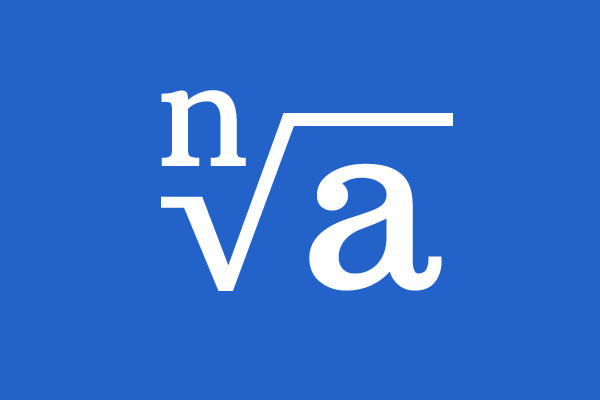# Surds## Definition

A positive integer root of a rational number is called a surd or a radical.

The irrational numbers are very special real numbers and no finite number of digits can represent them. However, some types of irrational numbers can be expressed in various roots of rational numbers and this type of representing an irrational number as a root of a rational number is called a surd.

### Example

$(1) \,\,\,\,\,$ $1.41421356237309504 \cdots$

$1.41421356237309504 \cdots$ is an irrational number. It is not possible to write it as a rational number and the value of this number cannot be written in number system completely. However, it can be written as a square root of the number $2$.

$\sqrt{2} = 1.41421356237309504 \cdots$

Observe the following few more examples for your better understanding.

$(2) \,\,\,\,\,$ $\sqrt[\displaystyle 3]{5} = 1.70997594667669 \cdots$

$(3) \,\,\,\,\,$ $\sqrt[\displaystyle 4]{2} = 1.18920711500272 \cdots$

$(4) \,\,\,\,\,$ $\sqrt[\displaystyle 5]{121} = 2.60949863527887 \cdots$

$(5) \,\,\,\,\,$ $\sqrt[\displaystyle 6]{7} = 1.38308755426848 \cdots$

In this way, some irrational numbers are written in simple form.

#### Algebraic form

If $n$ is a positive integer and $a$ is a rational number then a surd is written in algebraic form.

$\sqrt[\displaystyle n]a$

The value of $n$ is a positive integer and the value of $a$ is a position rational number. Therefore, the value of the surd is always positive.

Latest Math Topics
Jun 26, 2023
Jun 23, 2023

Latest Math Problems
Jul 01, 2023
Jun 25, 2023
###### Math Questions

The math problems with solutions to learn how to solve a problem.

Learn solutions

Practice now

###### Math Videos

The math videos tutorials with visual graphics to learn every concept.

Watch now

###### Subscribe us

Get the latest math updates from the Math Doubts by subscribing us.